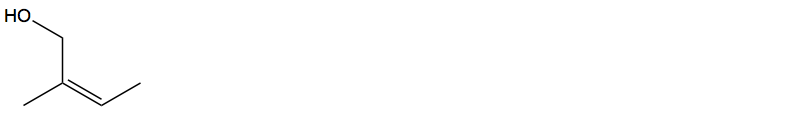# Problem: Determine the molecular formula corresponding to the following structure. Calculate the number of degrees of unsaturation from the molecular formula and evaluate whether your calculations agree with the structure shown.

🤓 Based on our data, we think this question is relevant for Professor Markworth's class at WWU.

###### Problem Details

Determine the molecular formula corresponding to the following structure. Calculate the number of degrees of unsaturation from the molecular formula and evaluate whether your calculations agree with the structure shown.What scientific concept do you need to know in order to solve this problem?

Our tutors have indicated that to solve this problem you will need to apply the Degrees of Unsaturation concept. You can view video lessons to learn Degrees of Unsaturation. Or if you need more Degrees of Unsaturation practice, you can also practice Degrees of Unsaturation practice problems.

What is the difficulty of this problem?

Our tutors rated the difficulty ofDetermine the molecular formula corresponding to the followi...as medium difficulty.

How long does this problem take to solve?

Our expert Organic tutor, Chris took 3 minutes and 49 seconds to solve this problem. You can follow their steps in the video explanation above.

What professor is this problem relevant for?

Based on our data, we think this problem is relevant for Professor Markworth's class at WWU.